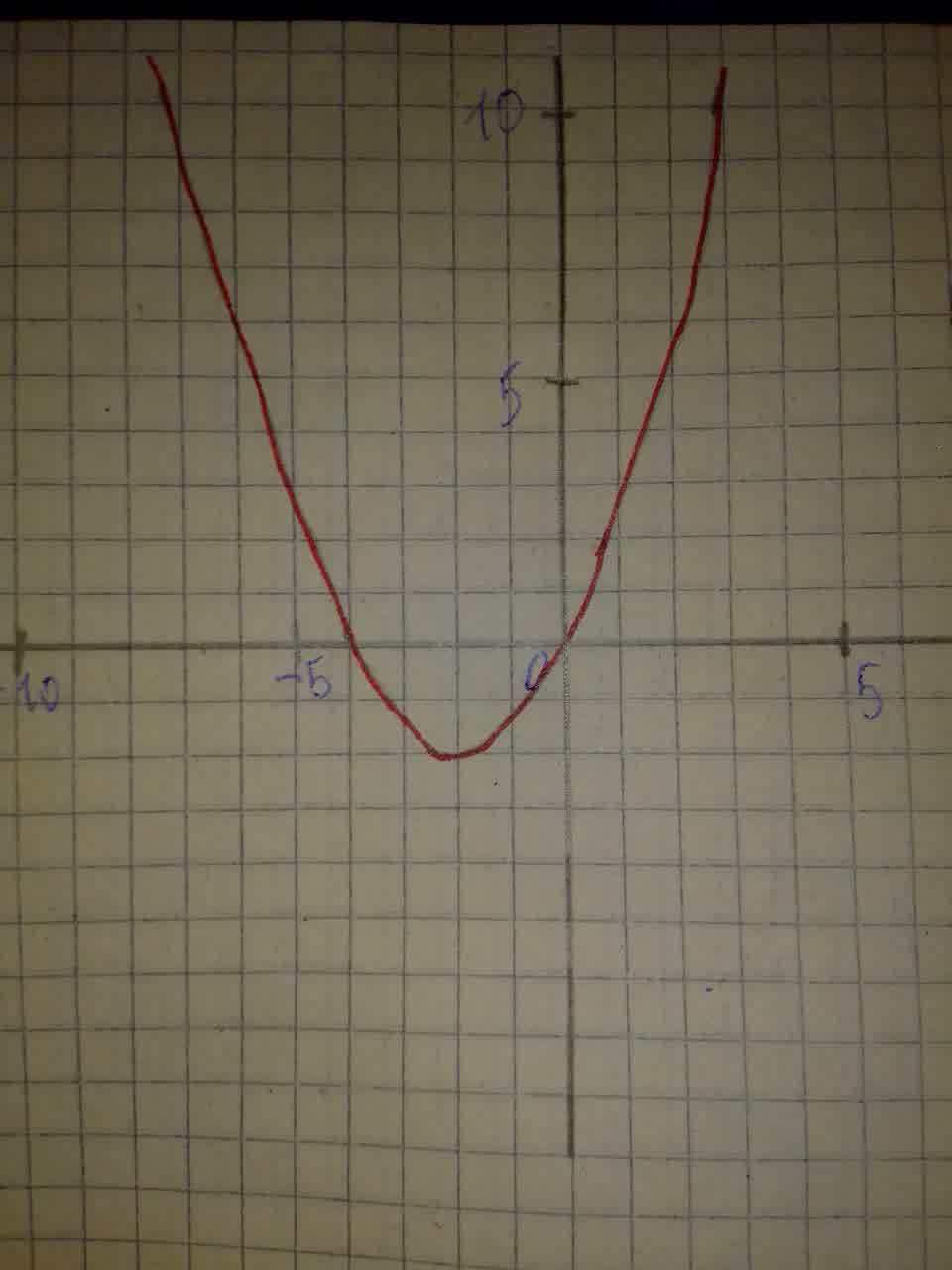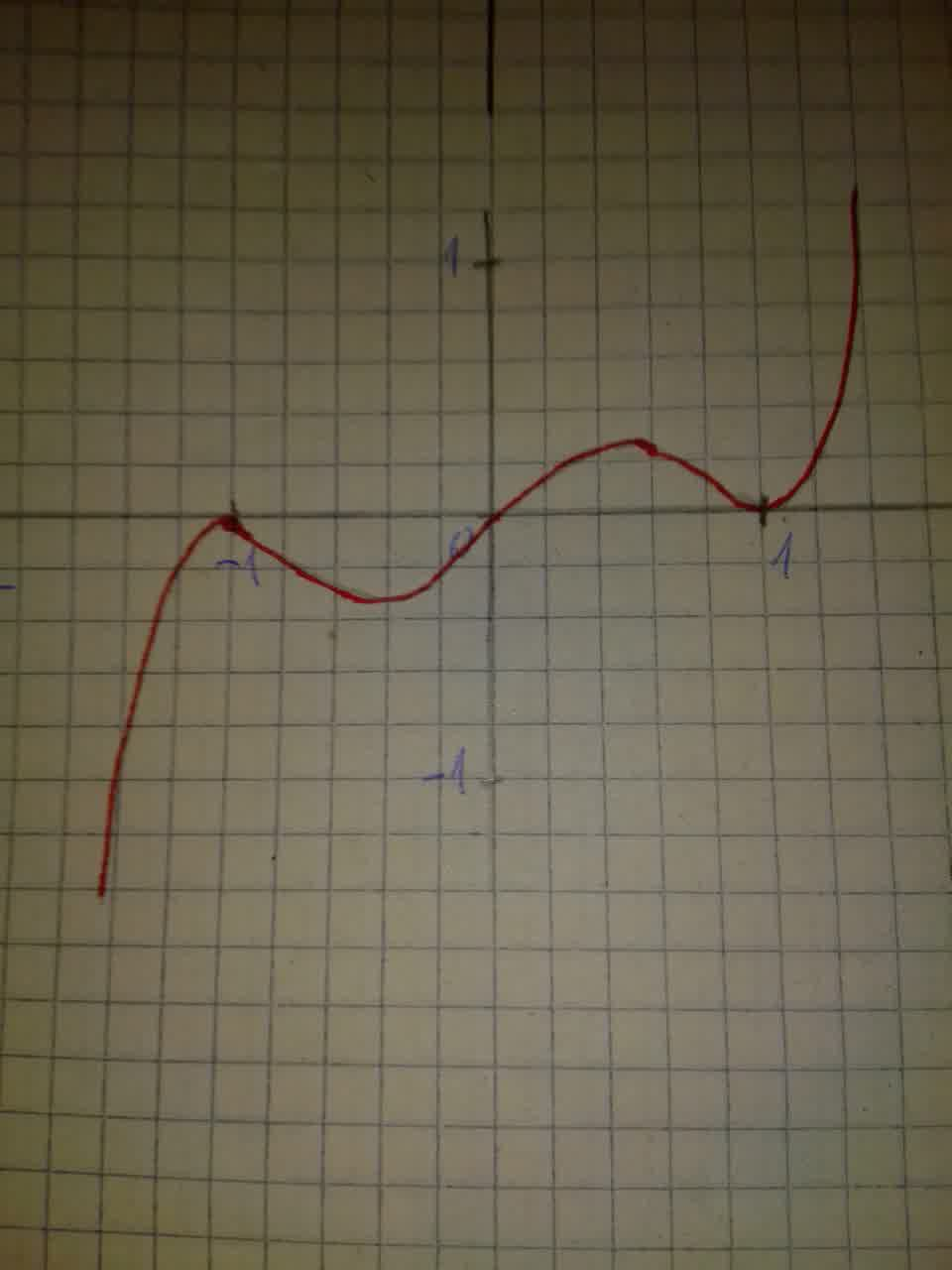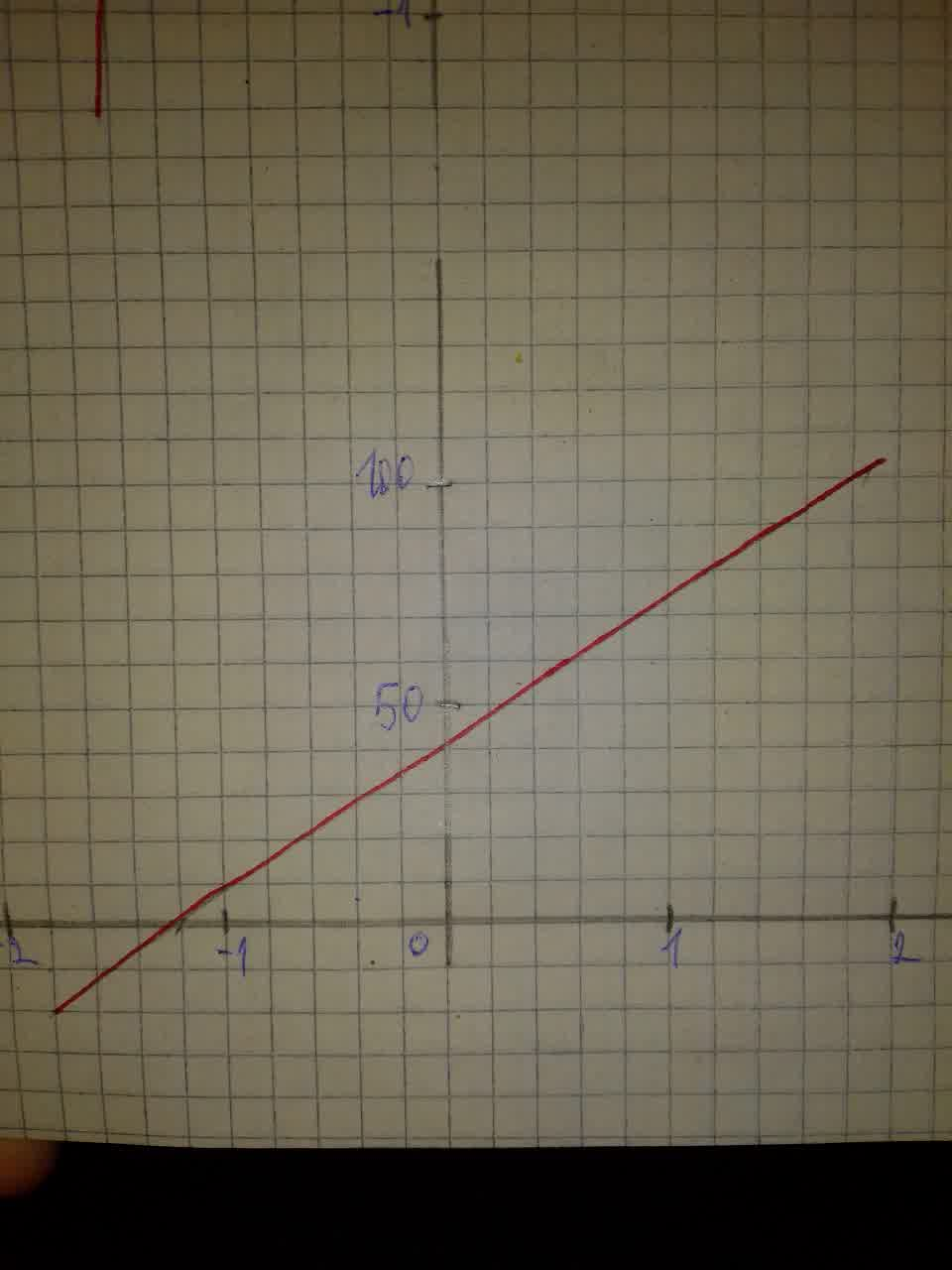# Use a graphing calculator to examine the graphs of the following functions a.f(x)=\frac{1}{2}x^{2}+2x\ b.f(x)=x(x+1)^{2}(x-1)^{2}\ c.f(x)=x^{3}-x^{2}+31x+40Anonym 2021-08-07 Answered
Use a graphing calculator to examine the graphs of the following functions. Determine ⋅⋅ the degree of the polynomial ⋅⋅ the leading coefficient ⋅⋅ the end behavior of the polynomial function ⋅⋅ the maximum number of zeros ⋅⋅ the maximum number of turning points (relative maxima and minima)
You can still ask an expert for help

• Questions are typically answered in as fast as 30 minutes

Solve your problem for the price of one coffee

• Math expert for every subject
• Pay only if we can solve itun4t5o4v

Step 1
a.Degree:2(highest-degree monomial is $\frac{1}{2}{x}^{2}$).
Leading coefficient: $\frac{1}{2}$(coefficient of highest-degree monomial)
End behavior: (even-degree polynomial with positive leading coefficient)
Maximum number of zeros: 2(same as degree)
Maximum number of turning points: 1(one less than degree)Step 2
b. Degree:5(product of 5 linear factors)
Leading coefficient: 1(product of coefficient of x terms)
End behavior: y (odd-degree polynomial with positive leading coefficient)
Maximum number of zeros: 5(same as degree)
Maximum number of turning points: 4 (one less than degree)Step 3
c. Degree: 3 (degree of highest-degree monomial ${x}^{3}$)
Leading coefficient: 1(coefficient of highest-degree monomial)
End behavior: (odd-degree polynomial with positive leading coefficient)
Maximum number of zeros: 3 (same as degree)
Maximum number of turning points: 2 (one less than degree)